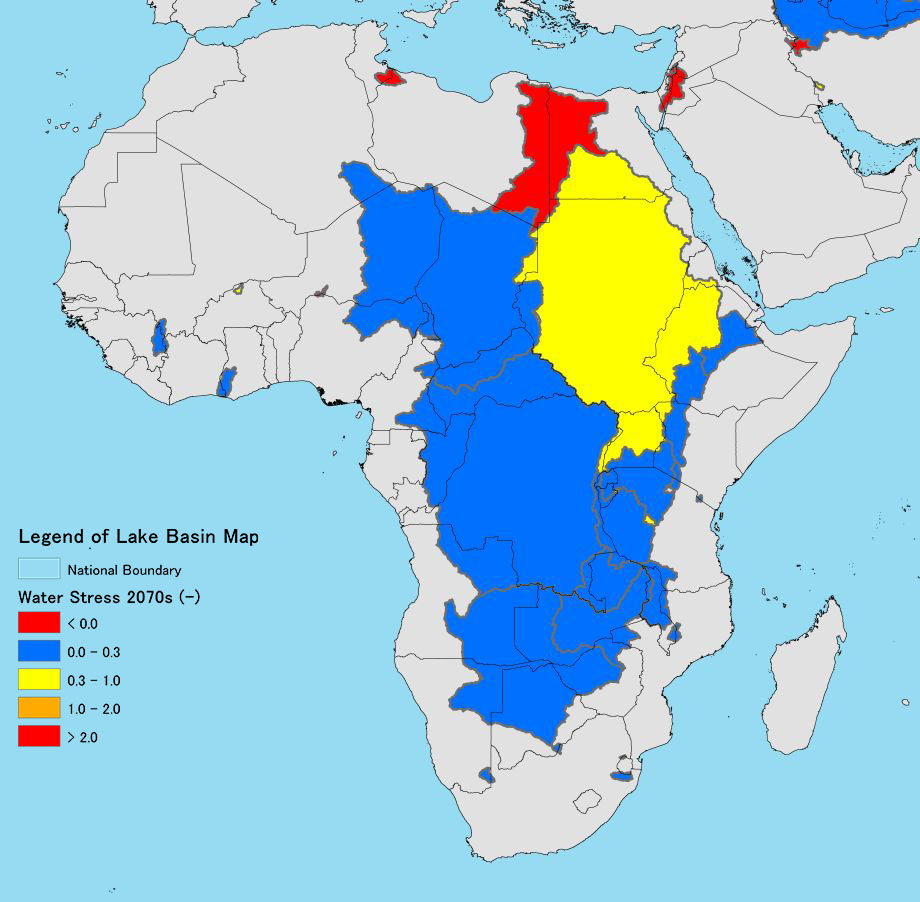The 2070 Water Stress was calculated by dividing the Water Demand by the Water Storage.

(The Water Storage was calculated using the 2070s value, and adding the monthly value to the annual value. The monthly water storage was calculated by subtracting the actual evapotranspiration from precipitation Volume. The actual evapotranspiration here was calculated by multiplying the possible evapotranspiration, based on monthly mean temperatures, by the evapotranspiration ratio. The Penman formula was used for the possible evapotranspiration, with the evapotranspiration ratio defined by the temperature designed for each climate class and the temperature-dependent regression equation).